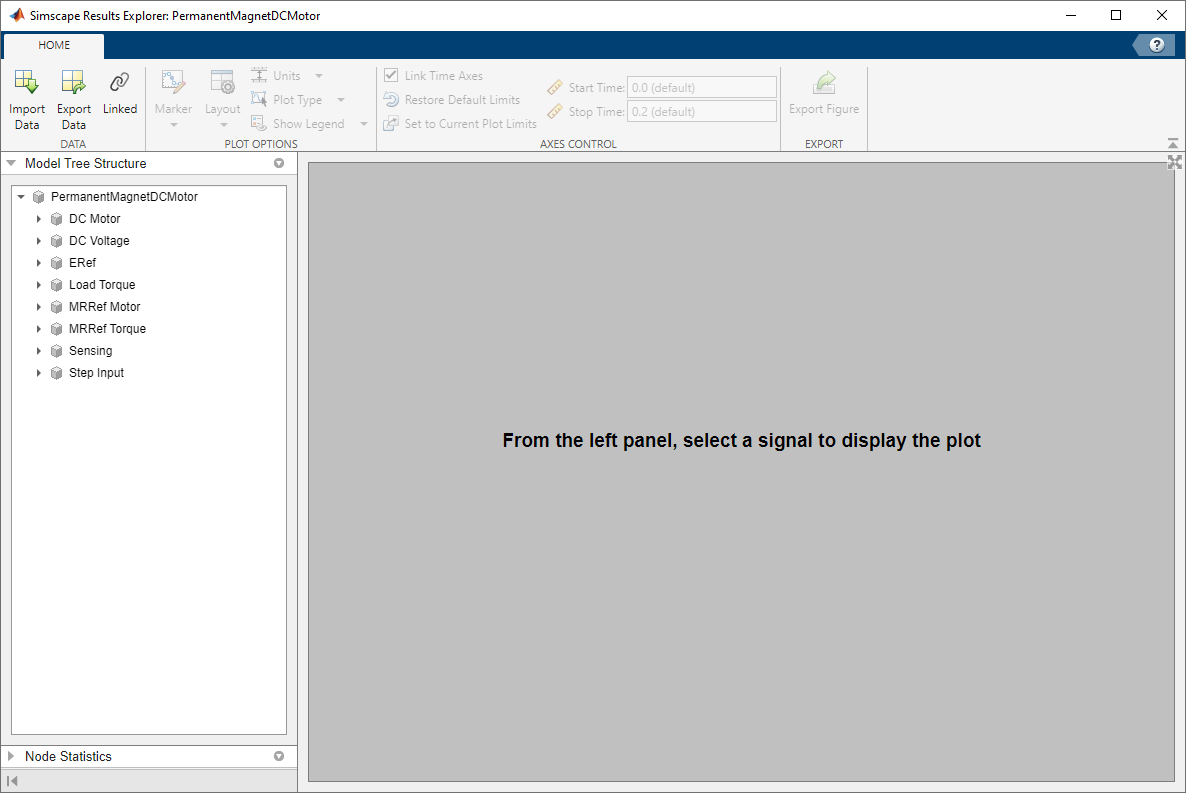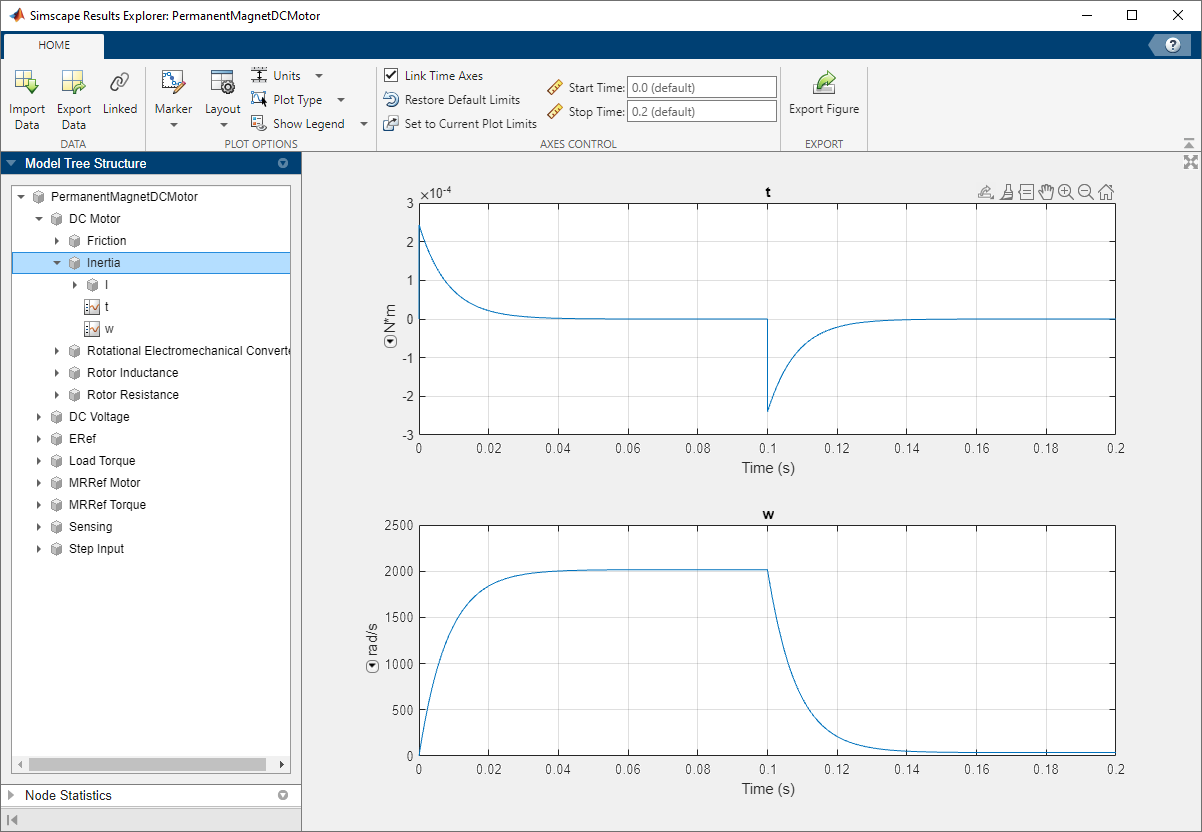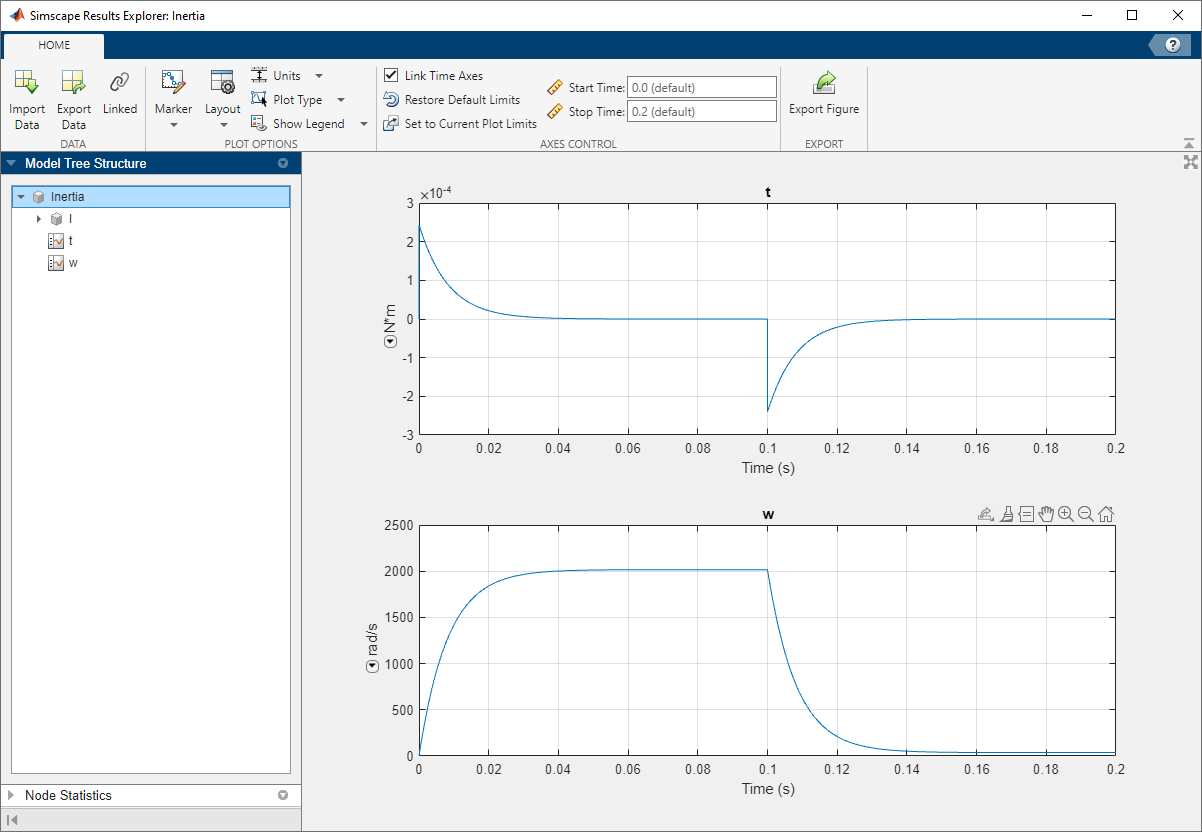# sscexplore

Open Simscape Results Explorer to interact with logged simulation data

## Syntax

``sscexplore(node)``
``sscexplore(node,nodepath)``

## Description

example

````sscexplore(node)` opens a new Simscape™ Results Explorer window containing logged simulation data for the specified node in a simulation log variable. Before you call this function, you must have the simulation log variable in your current workspace. Create the simulation log variable by simulating the model with data logging turned on, or load a previously saved variable from a file. If `node` is the name of the simulation log variable, then the Simscape Results Explorer window contains the data for the whole model. If `node` is the name of a node in the simulation data tree, then the Simscape Results Explorer window contains the data for that node only. ```

example

````sscexplore(node,nodepath)` opens a new Simscape Results Explorer window that contains logged simulation data for the specified node, `node`, but opens at a subnode specified by `nodepath`. ```

## Examples

collapse all

Open the Permanent Magnet DC Motor example model:

`openExample('simscape/PermanentMagnetDCMotorExample')`

This example model has data logging enabled for the whole model, with the Workspace variable name parameter set to `simlog_PermanentMagnetDCMotor`.

Simulate the model to log the simulation data:

`sim('PermanentMagnetDCMotor');`

Explore the simulation data:

`sscexplore(simlog_PermanentMagnetDCMotor)`

A new Simscape Results Explorer window opens. It contains logged simulation data for the whole model.As you expand and select nodes in the left pane, the corresponding plots appear in the right pane.

Open the Permanent Magnet DC Motor example model:

`openExample('simscape/PermanentMagnetDCMotorExample')`

This example model has data logging enabled for the whole model, with the Workspace variable name parameter set to `simlog_PermanentMagnetDCMotor`.

Simulate the model to log the simulation data:

`sim('PermanentMagnetDCMotor');`

Explore the simulation data:

`sscexplore(simlog_PermanentMagnetDCMotor,'DC_Motor.Inertia')`

A new Simscape Results Explorer window opens. It contains logged simulation data for the whole model, but the data exploration starts with the node specified by the `nodepath` argument. This node corresponds to the Inertia block in the DC Motor subsystem.Open the Permanent Magnet DC Motor example model:

`openExample('simscape/PermanentMagnetDCMotorExample')`

This example model has data logging enabled for the whole model, with the Workspace variable name parameter set to `simlog_PermanentMagnetDCMotor`.

Simulate the model to log the simulation data:

`sim('PermanentMagnetDCMotor');`

Explore the simulation data:

`sscexplore(simlog_PermanentMagnetDCMotor.DC_Motor.Inertia)`

A new Simscape Results Explorer window opens. It contains just the logged simulation data for the node specified in the `node` argument. This node corresponds to the Inertia block in the DC Motor subsystem.## Input Arguments

collapse all

Simulation log workspace variable, or a node within this variable, that contains the logged model simulation data, specified as a `Node` object. You specify the name of the simulation log variable by using the Workspace variable name parameter on the Simscape pane of the Configuration Parameters dialog box. To specify a node within the simulation log variable, provide the complete path to that node through the simulation data tree, starting with the top-level variable name.

Example: `simlog.DC_Motor.Motor_Inertia_J`

Path to a subnode to open, specified as a character vector or string scalar. If you omit the `nodepath` argument, then the Simscape Results Explorer window opens at the root of the specified node, `node`. If you specify `nodepath`, then the Simscape Results Explorer window still contains all the simulation data for the `node`, but opens at the subnode within it, specified by the `nodepath`. Use the `simscape.logging.findPath` function to find the `nodepath` value for a block or subsystem.

Example: `'DC_Motor.Motor_Inertia_J'`

Data Types: `char` | `string`

## Version History

Introduced in R2015a